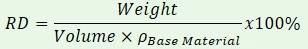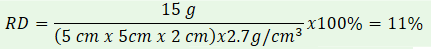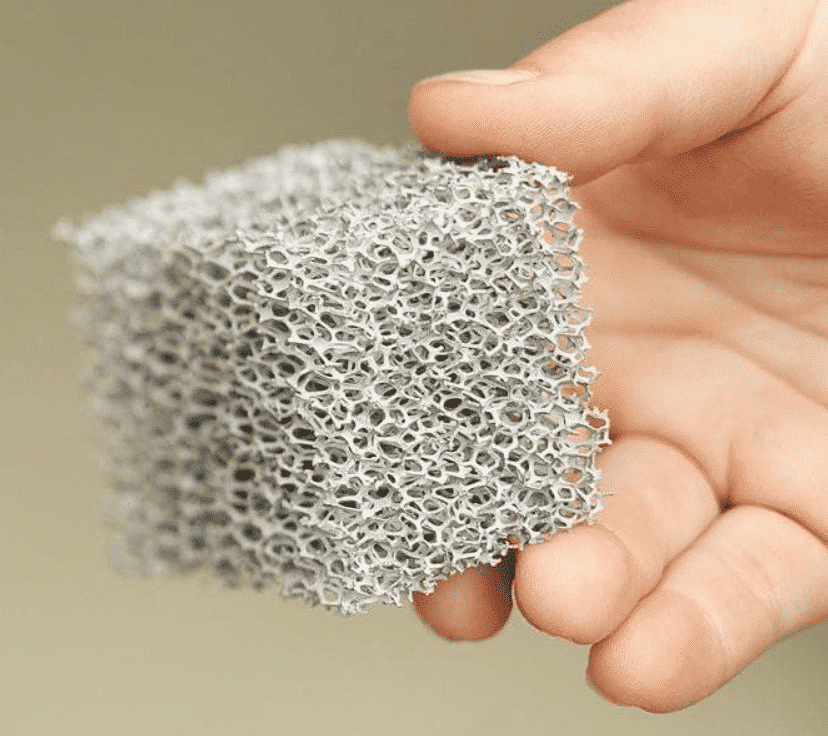# How is metal foam density calculated?

To calculate the density of metal or other rigid foams, measure the part to find the volume, weigh the part, then compare it to the weight of the part if the volume was solid material.  We have a few examples below.

### Why is foam density important?

Foam density is a measure of how much of a volume is taken up by the structure by weight. Density helps define the material requirements and controls the final weight of the part. For metal foams, the relative density directly correlates to the strength of the structure. This is crucial for designing a component like a crush structure, where the impact conditions require a specific strength that can be specified using the relative density of the metal foam.  See a more in depth discussion on foam strength on our energy absorption page

### How do you calculate foam density?

Foam is usually specified using relative density. Relative density represents the percentage of a given volume that is taken up by the foam structure. In other words, it’s a ratio of how much of the volume is occupied by the foam structure. Many applications prefer to specify porosity, or how much air is in the volume,  and relative density is the inverse of porosity, so porosity is defined as 1-RD.The simple way to measure the relative density is to measure the part to find the volume, weigh the part, then compare it to the weight of the part if the volume was solid material. Relative density is calculated by the following:For example, a 5 cm x 5 cm x 2 cm block of 6101 aluminum foam with a weight of 15 grams would have a relative density of 11%, and a porosity of 89%.Typical density ranges for Duocel® foams are:

• Metal foam = 4% to 12%, higher densities available for compressed or custom parts
• Carbon foam = 3% to 4%
• Ceramic foam = 4% to 20%